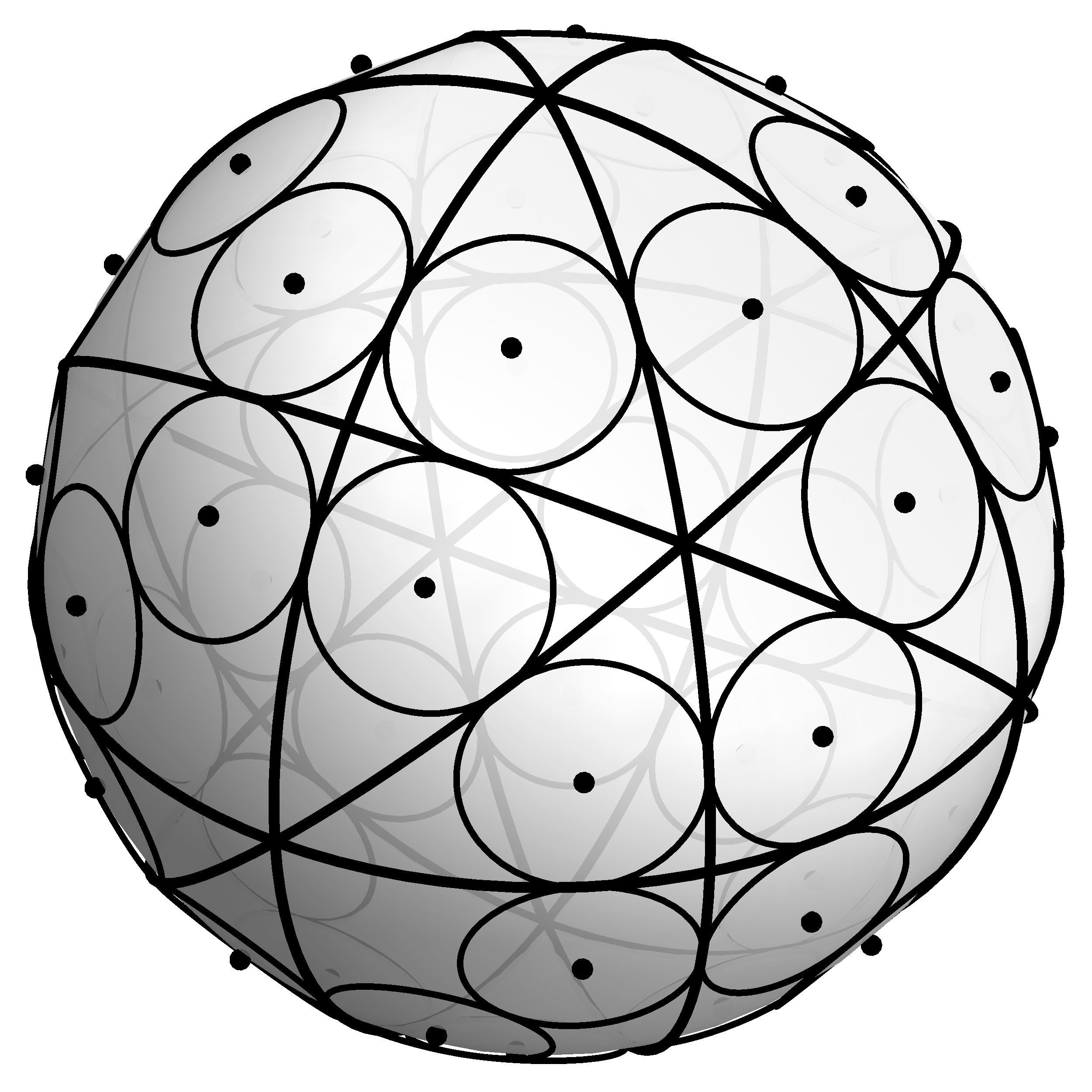# Clayton ShonkwilerIn our paper “Spherical geometry and the least symmetric triangle”, Laney Bowden, Andrea Haynes, Aaron Shukert, and I used the Grassmannnian model of triangle space to try to answer the question: “What is the least symmetric triangle?”

In this setting, the relevant Grassmannian is just $$\mathbb{RP}^2$$, which we view as double-covered by the standard unit sphere in 3-dimensional space, so we can take advantage of the spherical geometry to help us answer this question.

Specifically, since the triangles with a mirror symmetry are the isosceles triangles and the degenerate triangles, we just need to describe them as a subset of the sphere, then find the point(s) which are furthest from this subset. It turns out that the isosceles and degenerate triangles describe 9 great circles on the sphere which divide the sphere into 48 congruent spherical triangles. The incenters of these spherical triangles, as shown above, all correspond to congruent triangles which can justifiably be called the least symmetric triangle, shown below.